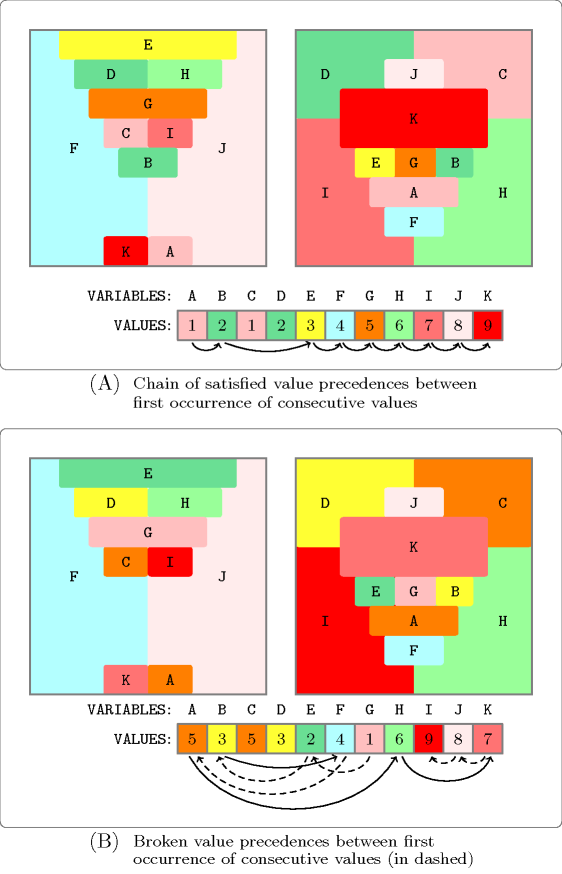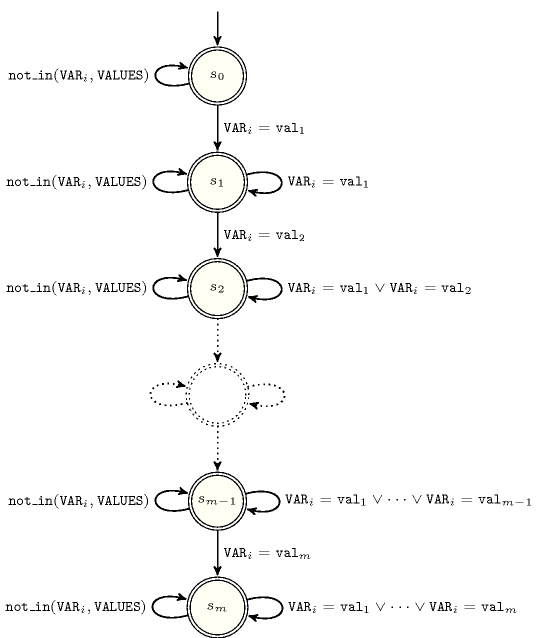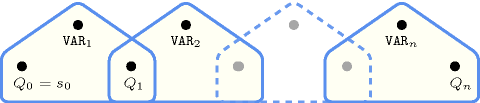## 5.196. int_value_precede_chain

Origin
Constraint

$\mathrm{𝚒𝚗𝚝}_\mathrm{𝚟𝚊𝚕𝚞𝚎}_\mathrm{𝚙𝚛𝚎𝚌𝚎𝚍𝚎}_\mathrm{𝚌𝚑𝚊𝚒𝚗}\left(\mathrm{𝚅𝙰𝙻𝚄𝙴𝚂},\mathrm{𝚅𝙰𝚁𝙸𝙰𝙱𝙻𝙴𝚂}\right)$

Synonyms

$\mathrm{𝚙𝚛𝚎𝚌𝚎𝚍𝚎}$, $\mathrm{𝚙𝚛𝚎𝚌𝚎𝚍𝚎𝚗𝚌𝚎}$, $\mathrm{𝚟𝚊𝚕𝚞𝚎}_\mathrm{𝚙𝚛𝚎𝚌𝚎𝚍𝚎}_\mathrm{𝚌𝚑𝚊𝚒𝚗}$.

Arguments
 $\mathrm{𝚅𝙰𝙻𝚄𝙴𝚂}$ $\mathrm{𝚌𝚘𝚕𝚕𝚎𝚌𝚝𝚒𝚘𝚗}\left(\mathrm{𝚟𝚊𝚛}-\mathrm{𝚒𝚗𝚝}\right)$ $\mathrm{𝚅𝙰𝚁𝙸𝙰𝙱𝙻𝙴𝚂}$ $\mathrm{𝚌𝚘𝚕𝚕𝚎𝚌𝚝𝚒𝚘𝚗}\left(\mathrm{𝚟𝚊𝚛}-\mathrm{𝚍𝚟𝚊𝚛}\right)$
Restrictions
 $\mathrm{𝚛𝚎𝚚𝚞𝚒𝚛𝚎𝚍}$$\left(\mathrm{𝚅𝙰𝙻𝚄𝙴𝚂},\mathrm{𝚟𝚊𝚛}\right)$ $\mathrm{𝚍𝚒𝚜𝚝𝚒𝚗𝚌𝚝}$$\left(\mathrm{𝚅𝙰𝙻𝚄𝙴𝚂},\mathrm{𝚟𝚊𝚛}\right)$ $\mathrm{𝚛𝚎𝚚𝚞𝚒𝚛𝚎𝚍}$$\left(\mathrm{𝚅𝙰𝚁𝙸𝙰𝙱𝙻𝙴𝚂},\mathrm{𝚟𝚊𝚛}\right)$
Purpose

Assuming $n$ denotes the number of items of the $\mathrm{𝚅𝙰𝙻𝚄𝙴𝚂}$ collection, the following condition holds for every $i\in \left[1,n-1\right]$: When it is defined, the first occurrence of the ${\left(i+1\right)}^{th}$ value of the $\mathrm{𝚅𝙰𝙻𝚄𝙴𝚂}$ collection should be preceded by the first occurrence of the ${i}^{th}$ value of the $\mathrm{𝚅𝙰𝙻𝚄𝙴𝚂}$ collection.

Example
$\left(〈4,0,1〉,〈4,0,6,1,0〉\right)$

The $\mathrm{𝚒𝚗𝚝}_\mathrm{𝚟𝚊𝚕𝚞𝚎}_\mathrm{𝚙𝚛𝚎𝚌𝚎𝚍𝚎}_\mathrm{𝚌𝚑𝚊𝚒𝚗}$ constraint holds since within the sequence 4, 0, 6, 1, 0:

• The first occurrence of value 4 occurs before the first occurrence of value 0.

• The first occurrence of value 0 occurs before the first occurrence of value 1.

Typical
 $|\mathrm{𝚅𝙰𝙻𝚄𝙴𝚂}|>1$ $\mathrm{𝚜𝚝𝚛𝚒𝚌𝚝𝚕𝚢}_\mathrm{𝚒𝚗𝚌𝚛𝚎𝚊𝚜𝚒𝚗𝚐}$$\left(\mathrm{𝚅𝙰𝙻𝚄𝙴𝚂}\right)$ $|\mathrm{𝚅𝙰𝚁𝙸𝙰𝙱𝙻𝙴𝚂}|>|\mathrm{𝚅𝙰𝙻𝚄𝙴𝚂}|$ $\mathrm{𝚛𝚊𝚗𝚐𝚎}$$\left(\mathrm{𝚅𝙰𝚁𝙸𝙰𝙱𝙻𝙴𝚂}.\mathrm{𝚟𝚊𝚛}\right)>1$ $\mathrm{𝚞𝚜𝚎𝚍}_\mathrm{𝚋𝚢}$$\left(\mathrm{𝚅𝙰𝚁𝙸𝙰𝙱𝙻𝙴𝚂},\mathrm{𝚅𝙰𝙻𝚄𝙴𝚂}\right)$
Symmetry

An occurrence of a value of $\mathrm{𝚅𝙰𝚁𝙸𝙰𝙱𝙻𝙴𝚂}.\mathrm{𝚟𝚊𝚛}$ that does not occur in $\mathrm{𝚅𝙰𝙻𝚄𝙴𝚂}.\mathrm{𝚟𝚊𝚛}$ can be replaced by any other value that also does not occur in $\mathrm{𝚅𝙰𝙻𝚄𝙴𝚂}.\mathrm{𝚟𝚊𝚛}$.

Arg. properties
• Contractible wrt. $\mathrm{𝚅𝙰𝙻𝚄𝙴𝚂}$.

• Suffix-contractible wrt. $\mathrm{𝚅𝙰𝚁𝙸𝙰𝙱𝙻𝙴𝚂}$.

• Aggregate: $\mathrm{𝚅𝙰𝙻𝚄𝙴𝚂}\left(\mathrm{𝚒𝚍}\right)$, $\mathrm{𝚅𝙰𝚁𝙸𝙰𝙱𝙻𝙴𝚂}\left(\mathrm{𝚞𝚗𝚒𝚘𝚗}\right)$.

Usage

The $\mathrm{𝚒𝚗𝚝}_\mathrm{𝚟𝚊𝚕𝚞𝚎}_\mathrm{𝚙𝚛𝚎𝚌𝚎𝚍𝚎}_\mathrm{𝚌𝚑𝚊𝚒𝚗}$ constraint is useful for breaking symmetries in graph colouring problems. We set a $\mathrm{𝚒𝚗𝚝}_\mathrm{𝚟𝚊𝚕𝚞𝚎}_\mathrm{𝚙𝚛𝚎𝚌𝚎𝚍𝚎}_\mathrm{𝚌𝚑𝚊𝚒𝚗}$ constraint on all variables ${V}_{1},{V}_{2},\cdots ,{V}_{n}$ associated with the vertices of the graph to colour, where we state that the first occurrence of colour $i$ should be located before the first occurrence of colour $i+1$ within the sequence ${V}_{1},{V}_{2},\cdots ,{V}_{n}$.

Figure 5.196.1 illustrates the problem of colouring earth and mars from Thom Sulanke. Part (A) of Figure 5.196.1 provides a solution where the first occurrence of each value of $i$, $\left(i\in \left\{1,2,\cdots ,8\right\}\right)$ is located before the first occurrence of value $i+1$. This is obtained by using the following constraints:

$\left\{\begin{array}{c}𝙰\ne 𝙱,𝙰\ne 𝙴,𝙰\ne 𝙵,𝙰\ne 𝙶,𝙰\ne 𝙷,𝙰\ne 𝙸,𝙰\ne 𝙹,𝙰\ne 𝙺,\hfill \\ 𝙱\ne 𝙰,𝙱\ne 𝙲,𝙱\ne 𝙵,𝙱\ne 𝙶,𝙱\ne 𝙷,𝙱\ne 𝙸,𝙱\ne 𝙹,𝙱\ne 𝙺,\hfill \\ 𝙲\ne 𝙱,𝙲\ne 𝙳,𝙲\ne 𝙵,𝙲\ne 𝙶,𝙲\ne 𝙷,𝙲\ne 𝙸,𝙲\ne 𝙹,𝙲\ne 𝙺,\hfill \\ 𝙳\ne 𝙲,𝙳\ne 𝙴,𝙳\ne 𝙵,𝙳\ne 𝙶,𝙳\ne 𝙷,𝙳\ne 𝙸,𝙳\ne 𝙹,𝙳\ne 𝙺,\hfill \\ 𝙴\ne 𝙰,𝙴\ne 𝙳,𝙴\ne 𝙵,𝙴\ne 𝙶,𝙴\ne 𝙷,𝙴\ne 𝙸,𝙴\ne 𝙹,𝙴\ne 𝙺,\hfill \\ 𝙵\ne 𝙰,𝙵\ne 𝙱,𝙵\ne 𝙲,𝙵\ne 𝙳,𝙵\ne 𝙴,𝙵\ne 𝙶,𝙵\ne 𝙷,𝙵\ne 𝙸,𝙵\ne 𝙹,𝙵\ne 𝙺,\hfill \\ 𝙶\ne 𝙰,𝙶\ne 𝙱,𝙶\ne 𝙲,𝙶\ne 𝙳,𝙶\ne 𝙴,𝙶\ne 𝙵,𝙶\ne 𝙷,𝙶\ne 𝙸,𝙶\ne 𝙹,𝙶\ne 𝙺,\hfill \\ 𝙷\ne 𝙰,𝙷\ne 𝙱,𝙷\ne 𝙲,𝙷\ne 𝙳,𝙷\ne 𝙴,𝙷\ne 𝙵,𝙷\ne 𝙶,𝙷\ne 𝙸,𝙷\ne 𝙹,𝙷\ne 𝙺,\hfill \\ 𝙸\ne 𝙰,𝙸\ne 𝙱,𝙸\ne 𝙲,𝙸\ne 𝙳,𝙸\ne 𝙴,𝙸\ne 𝙵,𝙸\ne 𝙶,𝙸\ne 𝙷,𝙸\ne 𝙹,𝙸\ne 𝙺,\hfill \\ 𝙹\ne 𝙰,𝙹\ne 𝙱,𝙹\ne 𝙲,𝙹\ne 𝙳,𝙹\ne 𝙴,𝙹\ne 𝙵,𝙹\ne 𝙶,𝙹\ne 𝙷,𝙹\ne 𝙸,𝙹\ne 𝙺,\hfill \\ 𝙺\ne 𝙰,𝙺\ne 𝙱,𝙺\ne 𝙲,𝙺\ne 𝙳,𝙺\ne 𝙴,𝙺\ne 𝙵,𝙺\ne 𝙶,𝙺\ne 𝙷,𝙺\ne 𝙸,𝙺\ne 𝙹,\hfill \\ \mathrm{𝚒𝚗𝚝}_\mathrm{𝚟𝚊𝚕𝚞𝚎}_\mathrm{𝚙𝚛𝚎𝚌𝚎𝚍𝚎}_\mathrm{𝚌𝚑𝚊𝚒𝚗}\left(〈1,2,3,4,5,6,7,8,9〉,〈𝙰,𝙱,𝙲,𝙳,𝙴,𝙵,𝙶,𝙷,𝙸,𝙹,𝙺〉\right).\hfill \end{array}\right\$

Part (B) provides a symmetric solution where the value precedence constraints between the pairs of values $\left(1,2\right)$, $\left(2,3\right)$, $\left(4,5\right)$, $\left(7,8\right)$ and $\left(8,9\right)$ are all violated (each violation is depicted by a dashed arc).

##### Figure 5.196.1. Using the $\mathrm{𝚒𝚗𝚝}_\mathrm{𝚟𝚊𝚕𝚞𝚎}_\mathrm{𝚙𝚛𝚎𝚌𝚎𝚍𝚎}_\mathrm{𝚌𝚑𝚊𝚒𝚗}$ constraint for breaking symmetries in graph colouring problems; there is an arc between the first occurrence of value $v$ $\left(1\le v\le 8\right)$ in the sequence of variables $𝙰$, $𝙱$, $𝙲$, $𝙳$, $𝙴$, $𝙵$, $𝙶$, $𝙷$, $𝙸$, $𝙹$, $𝙺$, and the first occurrence of value $v+1$ (a plain arc if the corresponding value precedence constraint holds, a dashed arc otherwise)Remark

When we have more than one class of interchangeable values (i.e., a partition of interchangeable values) we can use one $\mathrm{𝚒𝚗𝚝}_\mathrm{𝚟𝚊𝚕𝚞𝚎}_\mathrm{𝚙𝚛𝚎𝚌𝚎𝚍𝚎}_\mathrm{𝚌𝚑𝚊𝚒𝚗}$ constraint for breaking value symmetry in each class of interchangeable values. However it was shown in [Walsh07] that enforcing arc-consistency for such a conjunction of $\mathrm{𝚒𝚗𝚝}_\mathrm{𝚟𝚊𝚕𝚞𝚎}_\mathrm{𝚙𝚛𝚎𝚌𝚎𝚍𝚎}_\mathrm{𝚌𝚑𝚊𝚒𝚗}$ constraints is NP-hard.

Algorithm

The 2004 reformulation [Beldiceanu04] associated with the automaton of the Automaton slot achieves arc-consistency since the corresponding constraint network is a Berge-acyclic constraint network. Later on, another formulation into a sequence of ternary sliding constraints was proposed by [Walsh06]. It also achieves arc-consistency for the same reason.

Systems

specialisation: $\mathrm{𝚒𝚗𝚝}_\mathrm{𝚟𝚊𝚕𝚞𝚎}_\mathrm{𝚙𝚛𝚎𝚌𝚎𝚍𝚎}$ ($\mathrm{𝚜𝚎𝚚𝚞𝚎𝚗𝚌𝚎}$ of at least 2 $\mathrm{𝚟𝚊𝚕𝚞𝚎𝚜}$ replaced by $\mathrm{𝚜𝚎𝚚𝚞𝚎𝚗𝚌𝚎}$ of 2 $\mathrm{𝚟𝚊𝚕𝚞𝚎𝚜}$).

Keywords
Automaton

Figure 5.196.2 depicts the automaton associated with the $\mathrm{𝚒𝚗𝚝}_\mathrm{𝚟𝚊𝚕𝚞𝚎}_\mathrm{𝚙𝚛𝚎𝚌𝚎𝚍𝚎}_\mathrm{𝚌𝚑𝚊𝚒𝚗}$ constraint. Let $n$ and $m$ respectively denote the number of variables of the $\mathrm{𝚅𝙰𝚁𝙸𝙰𝙱𝙻𝙴𝚂}$ collection and the number of values of the $\mathrm{𝚅𝙰𝙻𝚄𝙴𝚂}$ collection. Let ${\mathrm{𝚅𝙰𝚁}}_{i}$ be the ${i}^{th}$ variable of the $\mathrm{𝚅𝙰𝚁𝙸𝙰𝙱𝙻𝙴𝚂}$ collection. Let ${\mathrm{𝚟𝚊𝚕}}_{v}$ $\left(1\le v\le m\right)$ denote the ${v}^{th}$ value of the $\mathrm{𝚅𝙰𝙻𝚄𝙴𝚂}$ collection.

##### Figure 5.196.2. Automaton of the $\mathrm{𝚒𝚗𝚝}_\mathrm{𝚟𝚊𝚕𝚞𝚎}_\mathrm{𝚙𝚛𝚎𝚌𝚎𝚍𝚎}_\mathrm{𝚌𝚑𝚊𝚒𝚗}$ constraint (state ${s}_{i}$ means that (1) each value ${\mathrm{𝚟𝚊𝚕}}_{1},{\mathrm{𝚟𝚊𝚕}}_{2},\cdots ,{\mathrm{𝚟𝚊𝚕}}_{i}$ was already encountered at least once, and that (2) value ${\mathrm{𝚟𝚊𝚕}}_{i+1}$ was not yet found)##### Figure 5.196.3. Hypergraph of the reformulation corresponding to the automaton of the $\mathrm{𝚒𝚗𝚝}_\mathrm{𝚟𝚊𝚕𝚞𝚎}_\mathrm{𝚙𝚛𝚎𝚌𝚎𝚍𝚎}_\mathrm{𝚌𝚑𝚊𝚒𝚗}$ constraint (since all states of the automaton are accepting there is no restriction on the last variable ${Q}_{n}$)We now show how to construct such an automaton systematically. For this purpose let us first introduce some notations:

• Without loss of generality we assume that we have at least two values (i.e., $m\ge 2$).

• Let $𝒞$ be the set of values that can be potentially assigned to a variable of the $\mathrm{𝚅𝙰𝚁𝙸𝙰𝙱𝙻𝙴𝚂}$ collection, but which do not belong to the values of the $\mathrm{𝚅𝙰𝙻𝚄𝙴𝚂}$ collection (i.e., $𝒞=\left(\mathrm{𝑑𝑜𝑚}\left({\mathrm{𝚅𝙰𝚁}}_{1}\right)\cup \mathrm{𝑑𝑜𝑚}\left({\mathrm{𝚅𝙰𝚁}}_{2}\right)\cup \cdots \cup \mathrm{𝑑𝑜𝑚}\left({\mathrm{𝚅𝙰𝚁}}_{n}\right)-\left\{{\mathrm{𝚟𝚊𝚕}}_{1},{\mathrm{𝚟𝚊𝚕}}_{2},\cdots ,{\mathrm{𝚟𝚊𝚕}}_{m}\right\}=\left\{{w}_{1},{w}_{2},\cdots ,{w}_{|𝒞|}\right\}$.

The states and transitions of the automaton are respectively defined in the following way:

• We have $m+1$ states labelled ${s}_{0},{s}_{1},\cdots ,{s}_{m}$ from which ${s}_{0}$ is the initial state. All states are accepting states.

• We have the following three sets of transitions:

1. For all $v\in \left[0,m-1\right]$, a transition from ${s}_{v}$ to ${s}_{v+1}$ labelled by value ${\mathrm{𝚟𝚊𝚕}}_{v+1}$. Each transition of this type will be triggered on the first occurrence of value ${\mathrm{𝚟𝚊𝚕}}_{v+1}$ within the variables of the $\mathrm{𝚅𝙰𝚁𝙸𝙰𝙱𝙻𝙴𝚂}$ collection.

2. For all $v\in \left[1,m\right]$ and for all $w\in \left[1,v\right]$, a self loop on ${s}_{v}$ labelled by value ${\mathrm{𝚟𝚊𝚕}}_{w}$. Such transitions encode the fact that we stay in the same state as long as we have a value that was already encountered.

3. If the set $𝒞$ is not empty, then for all $v\in \left[0,m\right]$ a self loop on ${s}_{v}$ labelled by the fact that we take a value not in $\mathrm{𝚅𝙰𝙻𝚄𝙴𝚂}$ (i.e., a value in $𝒞$). This models the fact that, encountering a value that does not belong to the set of values of the $\mathrm{𝚅𝙰𝙻𝚄𝙴𝚂}$ collection, leaves us in the same state.